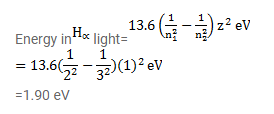# The light emitted in the transition n=3 to n=2 in hydrogen is called Ha light.

Question:

The light emitted in the transition $\mathrm{n}=3$ to $\mathrm{n}=2$ in hydrogen is called $\mathrm{H}_{\mathrm{a}}$ light. Find the maximum work function a metal can have so that $\mathrm{H}_{\mathrm{a}}$ light can emit photoelectrons from it.

Solution: